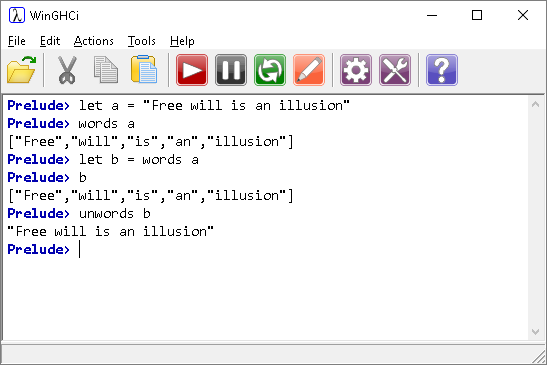## Introduction

In Haskell, a list is a data structure that can store multiple items of the same type. For example, a list of integers or a list of characters. A string in Haskell is considered to be a list of characters.

Square brackets are used to define a list. Notice the use of the let keyword when we want to introduce an identifier in the REPL terminal. Notice also that, by typing the identifier, we get the information associated with it,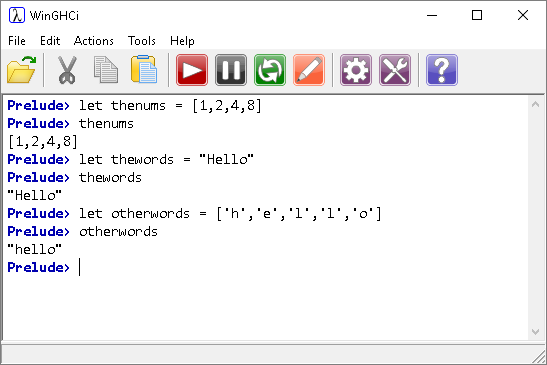The rest of this page runs through some of the built-in functions that you can use with lists.

## Empty List

Empty square brackets are used to denote an empty list.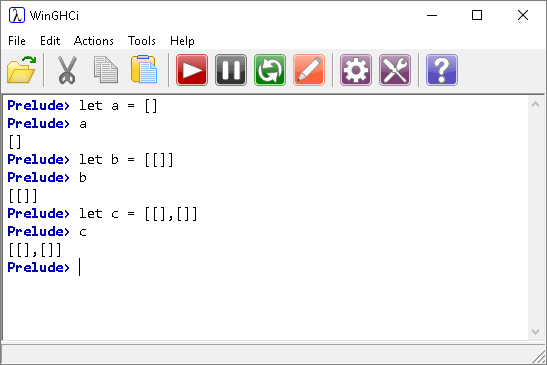In this example, a is an empty list, b is a list consisting of one item - an empty list. Finally, c is a list that consists of two items - two empty lists. These three things are not considered to be equal in Haskell.

## Concatenation (Appending)

The ++ operator is used for concatenating lists.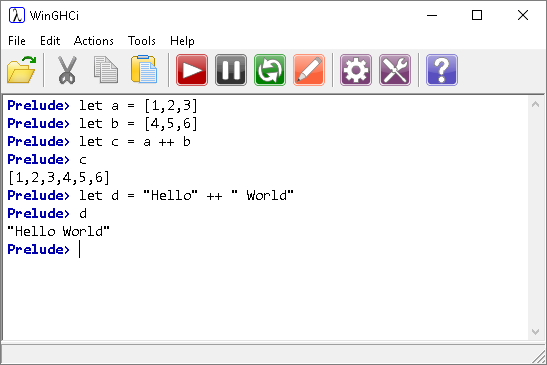The order of the list items is determined through construction. Consider the following,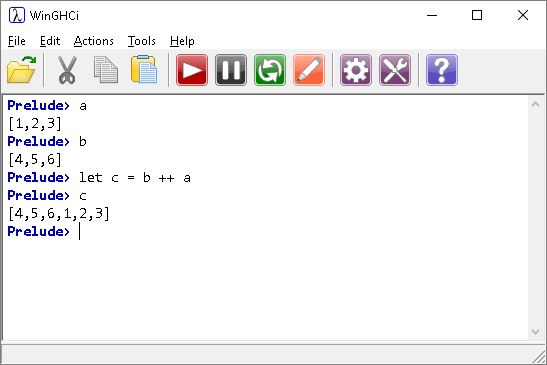When we concatenate, we are adding a list onto the beginning of another list.

a ++ b means add list a onto the front of list b. The important thing about this function is that it must be used with 2 lists. For example, this does not work,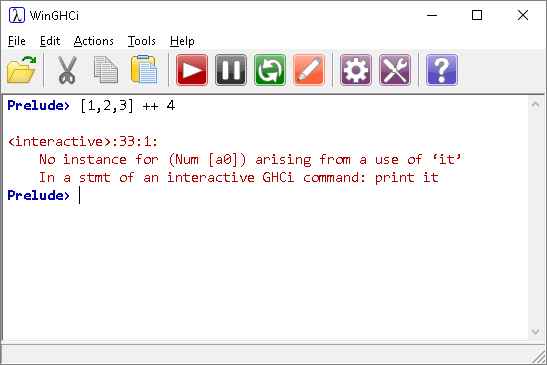Strings, being lists of characters can be concatenated using this function.

## Prepend

To prepend is to add to the start of a list. Study the following examples to see how that works.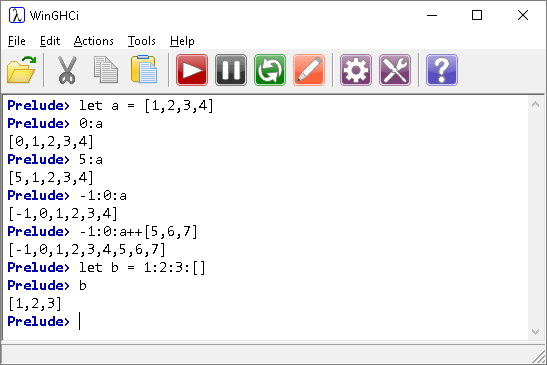The head function returns the first item in a list.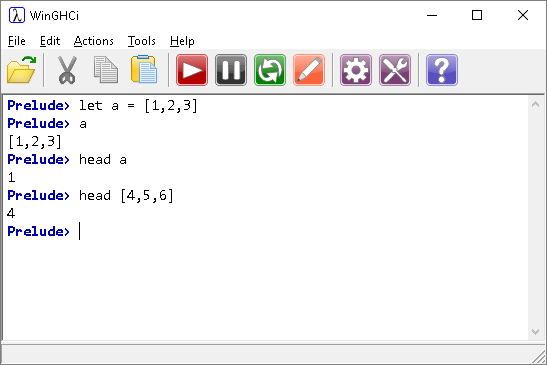The tail function returns all elements in a list except the first one.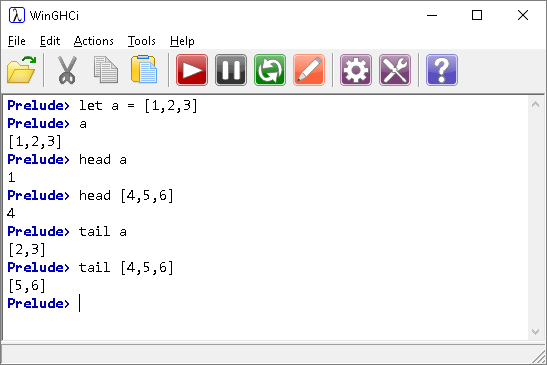The tail of a list of length 1 would be an empty list. Study the following example.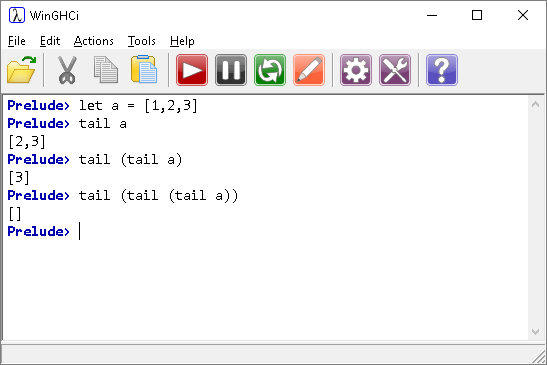## Last & Init

The last function is the opposite of the head function. It returns the last element in a list. The init function is the opposite of the tail function. It returns all elements from a list except the last one.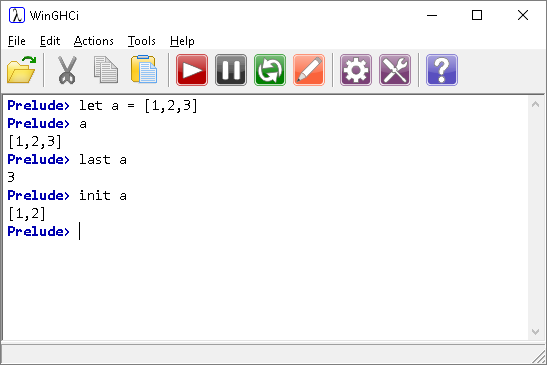## List Length

The length function returns the length of a list.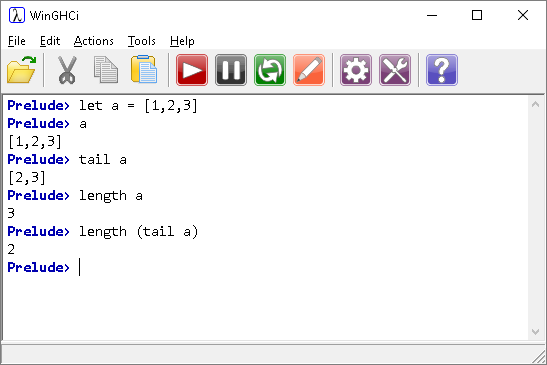## Empty List

You can use the null function to check if a list is empty.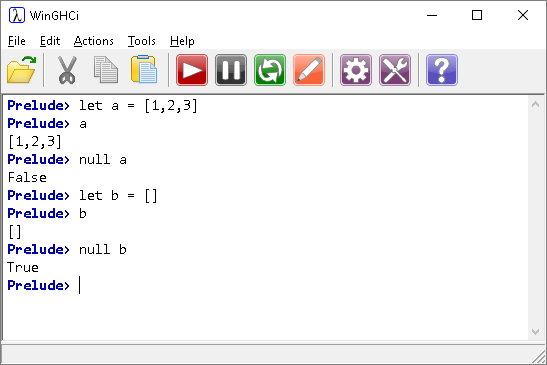## Accessing Items In A List

The !! operator returns an item from a list based on its zero-indexed position.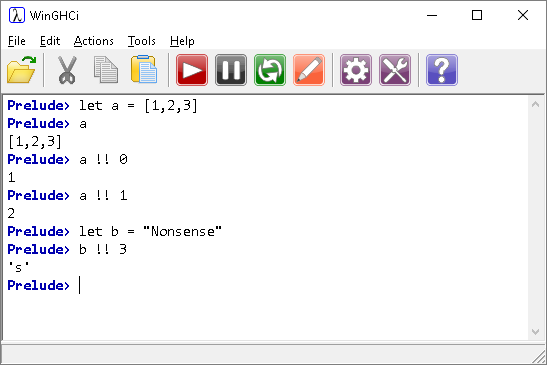## Sum & Product

The sum and product functions do exactly what you expect them to do with a list.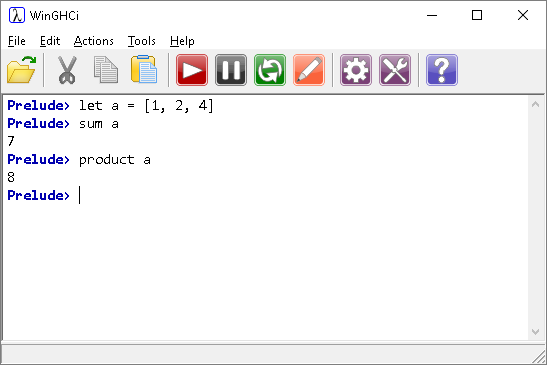## Reverse

Again, as you might expect.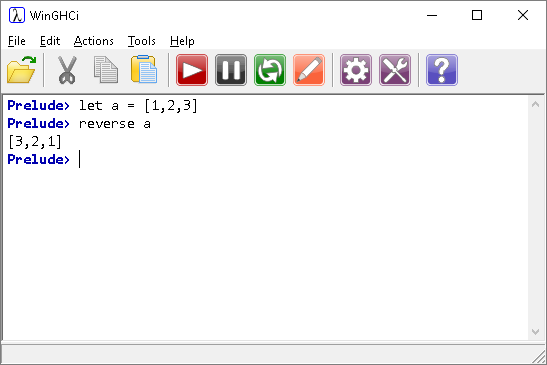## Concat

This is useful for turning a list of lists into a single list. For example,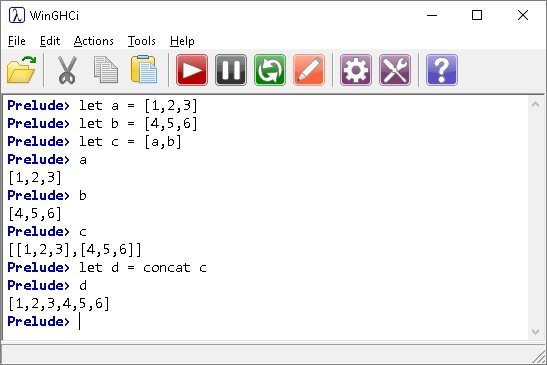## In The List Or Not

We can tell whether an item is an element of a list by using the elem and notElem functions.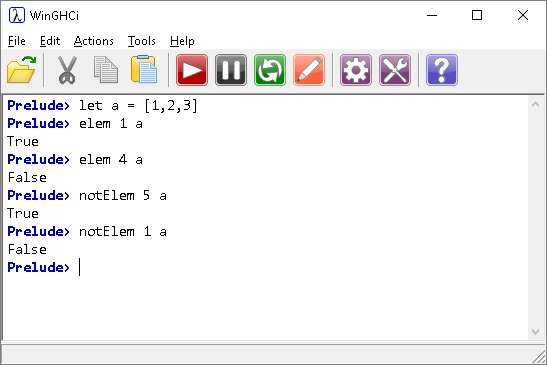## Taking & Dropping

The take function gives you the first n elements of a list. The drop function gives you everything except the first n elements.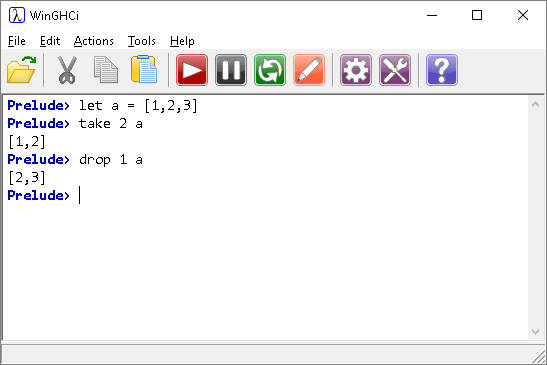## Words & Unwords

The words function will separate a string by its spaces. The unwords function does the opposite.